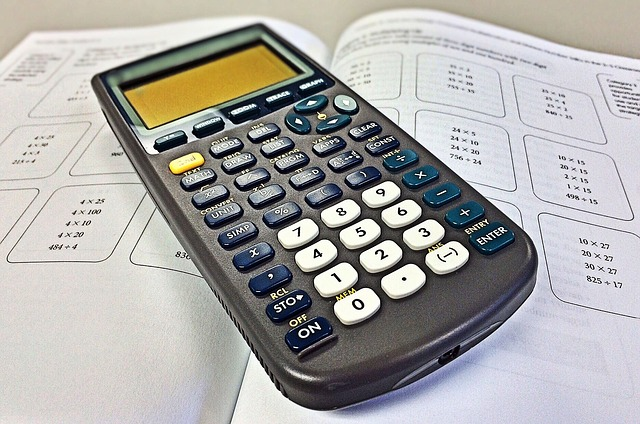The Easiest Ways To Calculate Fractions

What Are Fractions?

A fractional number, or fraction, is used to represent a segment of a whole number.

A fraction will include two numbers, one above the other. The first number, found above the line, is the numerator. The second number, located below the line, is the denominator.

A denominator indicates the total number of equal parts into which something is divided. The numerator shows how many of these equal parts need to be taken into consideration.

An easy way to remember fractions is to refer to the line that separates each number as ‘out of’. So a fraction written as 3/5 simply refers to 3 pieces out of 5 equal sections.

How Can Fractions Be Presented?

Fractions can be presented in three ways: as proper fractions, improper fractions and mixed number fractions.

• A proper fraction is one where the numerator is less than the denominator. For example, ⅔ (two-thirds) or ⅞ (seven-eighths).
• An improper fraction has a larger numerator than denominator. For example, 8/5 (eight-fifths) or 13/4 (thirteen quarters).
• A mixed number combines a whole number and a fraction. For example, 5¾ (five and three-quarters) or 12⅖ (twelve and two-fifths).Simplifying Fractions

The process of simplifying fractions reduces them to their simplest form. As an example, it’s much easier to refer to something as ½ rather than 4/8.

There are two ways in which to simplify a fraction.

The first method is to equally divide the top and bottom of the fraction by whole numbers larger than 1 until you cannot go any further. As an example, let’s take the fraction 24/108:

• Divide each number by 2 to get 12/54
• Divide by 2 again to get 6/27
• Divide by 3 to get 2/9

To add fractions together, you’ll need to modify them so that the denominators (bottom numbers) are the same. Then you total the numerators.

Let’s say that you wanted to add the fraction ¼ to ¼.

The denominators are already the same, so you can go to the second step and add 1 to 1.

The second half of the fraction stays the same, so adding the fractions ¼ and ¼ equals 2/4 (or ½).

Say you want to add the fractions ⅓ and ⅙.

To get the denominators to match, change ⅓ to 2/6.

Add 1 to 2 to get 3, and place 6 below. The answer is 3/6. Simplify that to ½.

Subtracting Fractions

Subtracting fractions works in a similar way:

• Step 1 – Ensure that the denominators are the same
• Step 2 – Subtract the numerators
• Step 3 – Simplify the fraction if required

Subtraction: Example 1

Let’s say you were asked to work out ¾ - ¼

The first step is relatively easy because the numbers are the same.

The second step involves subtracting the top numbers and then putting the answer over the same denominator.

So ¾ - ¼ would be worked out as 3 - 1 = 2

The answer would therefore be 2/4, which is ½.

Multiplying Fractions

Multiplying fractions is relatively easy; you simply multiply the top numbers and the bottom numbers.

If, for example, you multiply the fractions ½ and ⅓, you get ⅙. You are not expected to find the common denominator through multiplication.

Dividing Fractions

To divide fractions, you need to turn the fraction you are dividing by upside-down. As an example, if you wanted to divide ½ by ⅓, you rewrite the equation so the second fraction is 3/1. Then multiply ½ by 3/1, which leaves you with 3/2.

It may be necessary to further reduce the fraction to reach a compound fraction.

Common Mistakes and Things to Look Out For

It can be easy to get overwhelmed when adding and subtracting fractions. Students will often add or subtract denominators or numerators of two fractions, and commonly fail to recognise the connection between the denominator. To further add to the confusion, numerators and denominators should be approached as whole numbers in the calculation, eg when you are required to multiply a fraction.

Let’s take an example, adding together ¾ and ⅙.

The first thing to do is get the denominators the same, so we multiply them to get 24.

We multiplied the denominator 4 by 6 to get 24, so we multiply the numerator by 6 also, to get 18/24.

We multiplied the denominator 6 by 4 to get 24, so we multiply the numerator by 4 also, to get 4/24.

Now we can simply add 18/24 to 4/24, to get 22/24, which simplifies to 11/12.

Other common mistakes include:

• When adding or subtracting fractions, candidates may forget to first convert fractions so that they have a common denominator.
• Changing the denominator of a fraction without completing the necessary changes to the numerator.
• Misunderstanding the question completely; eg dividing rather than subtracting, or multiplying rather than adding.
• Leaving the denominator unchanged during questions that relate to multiplication or addition.

Understanding the relation between mixed numbers and improper fractions, and how to translate each into the other, is crucial for working with fractions.